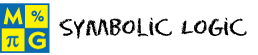# Symbolic LogicThis page lists the Learning Objectives for all lessons in Unit 9.

#### Negation

The student will be able to:

• Define closed sentence, open sentence, statement, negation, truth value and truth tables.
• Example examples in which a simple sentence is written in symbolic form.
• Determine if a sentence is true, false or open.
• Express the negation of a statement in symbolic form and in sentence form.
• Recognize that a statement and its negation have opposite truth values.
• Determine the truth values for a given statement and its negation.
• Construct a truth table to summarize truth values.
• Connect negation with written English.
• Apply negation concepts to complete five interactive exercises.

#### Conjunction

The student will be able to:

• Define logical connector, compound statement and conjunction.
• Express a conjunction in symbolic form and in sentence form.
• Recognize that the conjunction of two open sentences depends on the replacement value of the variable in each.
• Determine the truth values of a conjunction, given the truth values of each part.
• Construct a truth table for a conjunction to determine its truth values.
• Recognize that a truth table is an excellent tool for summarizing the truth values of statements.
• Integrate conjunction with other topics in mathematics.
• Apply conjunction concepts to complete five interactive exercises.

#### Disjunction

The student will be able to:

• Define disjunction.
• Express a disjunction in symbolic form and in sentence form.
• Recognize that the disjunction of two open sentences depends on the replacement value of the variable in each.
• Determine the truth values for a disjunction, given the truth values of each part.
• Construct a truth table for a disjunction to determine its truth values.
• Construct a truth table for the conjunction and disjunction of two statements.
• Distinguish between a disjunction and a conjunction.
• Integrate disjunction with other topics in mathematics.
• Apply disjunction concepts to complete five interactive exercises.

#### Conditional

The student will be able to:

• Define conditional statement, hypothesis and conclusion.
• Identify the hypothesis and conclusion of a conditional statement.
• Express a conditional statement in symbolic form and in sentence form.
• Construct a truth table for a conditional statement.
• Determine the truth value of the conditional, given the truth values of its hypothesis and conclusion.
• Integrate conditional statements with other topics in mathematics.
• Apply conditional concepts to complete five interactive exercises.

#### Compound Statements

The student will be able to:

• Define symbolic form.
• Examine sentences represented by compound statements with the connectors ~,,,and.
• Express compound statements in symbolic form with the connectors ~,,,and.
• Determine the truth value of a compound statement, given the truth values of each part.
• Construct a truth table for a compound statement, given in symbolic form, to determine its truth values.
• Integrate compound statements with other topics in mathematics.
• Apply compound statement concepts to complete five interactive exercises.

#### Biconditional

The student will be able to:

• Define biconditional statement.
• Given a hypothesis and a conclusion, construct a biconditional statement in sentence form
• Given a hypothesis and a conclusion, construct a biconditional statement in sentence in symbolic form.
• Given a hypothesis and a conclusion, construct a truth table for the biconditional statement.
• Express biconditional statements using "if and only if" or "iff".
• Explain the relationship between a conditional and a biconditional statement.
• Integrate biconditional statements with other topics in mathematics.
• Apply biconditional concepts to complete five interactive exercises.

#### Tautologies

The student will be able to:

• Review disjunction, negation and compound statements.
• Define tautology.
• Decipher the individual parts of a compound statement.
• Determine if a compound statement is a tautology by constructing a truth table for its individual parts.
• Apply tautology concepts to complete five interactive exercises.

#### Equivalent Statements

The student will be able to:

• Define logical equivalence.
• Construct a truth table for three compound statements to determine which two are logically equivalent.
• Recognize that the biconditional of two equivalent statements is a tautology.
• Apply equivalence concepts to complete five interactive exercises.

#### Practice Exercises

The student will be able to:

• Examine ten interactive exercises for all topics in this unit.
• Determine which concepts and procedures are needed to complete each practice exercise.
• Complete interactive truth tables by applying concepts and procedures from symbolic logic.
• Compute answers by applying concepts and procedures from symbolic logic.
• Self-assess knowledge and skills acquired from this unit.

#### Challenge Exercises

The student will be able to:

• Evaluate ten interactive exercises for all topics in this unit.
• Analyze each problem to identify the given information.
• Determine the truth values of compound statements.
• Apply logic concepts to solve complex problems.

#### Solutions

The student will be able to:

• Examine the solution for each exercise presented in this unit.
• Identify which solutions need to be reviewed.
• Compare solutions to completed exercises.
• Identify and evaluate incorrect answers.
• Repeat exercises that were incorrectly answered.
• Identify areas of strength and weakness.
• Decide which concepts and procedures need to be reviewed from this unit.

### Order your CD today for Home or SchoolOR